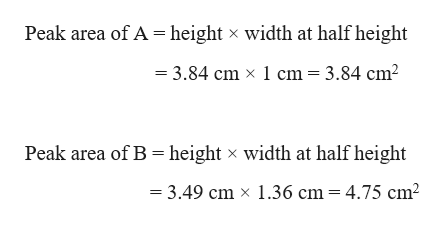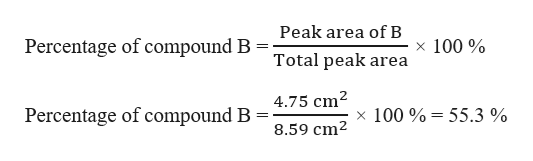# You have obtained a gas chromatogram of a two component mixture of compounds A and B. The height of peak A is 3.84 cm. and the width at half height is 1 cm. The height of peak B is 3.49 cm. and the width at half height is 1.36 cm. The percentage of a component in the mixture is proportional to peak area. Estimate peak areas by multiplying height by width at half height. What was the percentage of compound B in the mixture? (Enter your answer as a number without any percent symbol or the word percent.)

Question
1 views

You have obtained a gas chromatogram of a two component mixture of compounds A and B. The height of peak A is 3.84 cm. and the width at half height is 1 cm. The height of peak B is 3.49 cm. and the width at half height is 1.36 cm. The percentage of a component in the mixture is proportional to peak area. Estimate peak areas by multiplying height by width at half height. What was the percentage of compound B in the mixture? (Enter your answer as a number without any percent symbol or the word percent.)

check_circle

Step 1

Given,

Height of peak A = 3.84 cm

Width of A at half height = 1 cm

Height of peak B = 3.49 cm

Width of B at half height = 1.36 cm

The percentage of a component in the mixture is proportional to peak area. Peak areas is to be estimated by multiplying height by width at half height.

Step 2

Therefore, peak area of A and B can be calculated as:help_outlineImage TranscriptionclosePeak area of A height x width at half height 3.84 cm x 1 cm = 3.84 cm2 Peak area of B height width at half height x = 3.49 cm x 1.36 cm 4.75 cm2 fullscreen
Step 3

Total peak area = 3.84 cm2 + 4.75 cm2 = 8.59 cm2

The percentage of compound ...help_outlineImage TranscriptionclosePeak area of B Percentage of compound B x 100% Total peak area 4.75 cm2 Percentage of compound B x 100%55.3 % 8.59 cm2 fullscreen

### Want to see the full answer?

See Solution

#### Want to see this answer and more?

Solutions are written by subject experts who are available 24/7. Questions are typically answered within 1 hour.*

See Solution
*Response times may vary by subject and question.
Tagged in

### Other